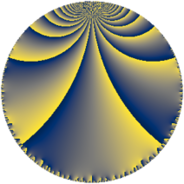# Properties

 Label 207.6.iLevel $207$ Weight $6$ Character orbit 207.i Rep. character $\chi_{207}(55,\cdot)$ Character field $\Q(\zeta_{11})$ Dimension $490$ Sturm bound $144$

# Related objects

## Defining parameters

 Level: $$N$$ $$=$$ $$207 = 3^{2} \cdot 23$$ Weight: $$k$$ $$=$$ $$6$$ Character orbit: $$[\chi]$$ $$=$$ 207.i (of order $$11$$ and degree $$10$$) Character conductor: $$\operatorname{cond}(\chi)$$ $$=$$ $$23$$ Character field: $$\Q(\zeta_{11})$$ Sturm bound: $$144$$

## Dimensions

The following table gives the dimensions of various subspaces of $$M_{6}(207, [\chi])$$.

Total New Old
Modular forms 1240 510 730
Cusp forms 1160 490 670
Eisenstein series 80 20 60

## Trace form

 $$490 q + 7 q^{2} - 819 q^{4} + 39 q^{5} - 29 q^{7} + 272 q^{8} + O(q^{10})$$ $$490 q + 7 q^{2} - 819 q^{4} + 39 q^{5} - 29 q^{7} + 272 q^{8} + 355 q^{10} - 1039 q^{11} - 735 q^{13} - 561 q^{14} - 9995 q^{16} + 2962 q^{17} + 4610 q^{19} - 3717 q^{20} - 9498 q^{22} - 9384 q^{23} - 18016 q^{25} + 8184 q^{26} + 27235 q^{28} + 23050 q^{29} - 5978 q^{31} - 35169 q^{32} + 83261 q^{34} - 25219 q^{35} - 77851 q^{37} + 55646 q^{38} + 33907 q^{40} + 281 q^{41} + 51757 q^{43} - 135536 q^{44} + 164869 q^{46} - 45222 q^{47} - 140802 q^{49} - 2404 q^{50} - 215936 q^{52} + 34663 q^{53} - 84277 q^{55} + 49010 q^{56} - 44411 q^{58} - 377799 q^{59} - 148353 q^{61} - 117448 q^{62} - 227262 q^{64} + 108904 q^{65} + 169665 q^{67} + 614718 q^{68} + 243566 q^{70} + 262754 q^{71} - 13145 q^{73} - 386428 q^{74} - 433630 q^{76} - 219404 q^{77} - 61647 q^{79} + 121330 q^{80} + 364354 q^{82} + 369534 q^{83} + 261341 q^{85} + 352439 q^{86} - 194823 q^{88} - 287089 q^{89} - 249052 q^{91} - 763276 q^{92} - 1041709 q^{94} - 775704 q^{95} - 224380 q^{97} + 73483 q^{98} + O(q^{100})$$

## Decomposition of $$S_{6}^{\mathrm{new}}(207, [\chi])$$ into newform subspaces

The newforms in this space have not yet been added to the LMFDB.

## Decomposition of $$S_{6}^{\mathrm{old}}(207, [\chi])$$ into lower level spaces

$$S_{6}^{\mathrm{old}}(207, [\chi]) \cong$$ $$S_{6}^{\mathrm{new}}(23, [\chi])$$$$^{\oplus 3}$$$$\oplus$$$$S_{6}^{\mathrm{new}}(69, [\chi])$$$$^{\oplus 2}$$